# 人民币转换大写

1. 人民币数字金额大小写转换器(将人民币阿拉伯数字转换成中文大写、人民币大写转换工具)。

## 阿拉伯金额数字对应中文大写表 - 数字转大写金额 (以下红色字可选中复制)

•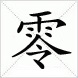0 =
•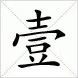1 =
•2 =
•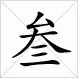3 =
•4 =
•5 =
•6 =
•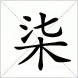7 =
•8 =
•9 =

## 金额单位(位数)对应表

•亿 = 亿
•万 =
•千 =
•百 =
•十 =
•元 = 元(圆)
•角 =
•分 =
•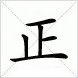正 =
•整 =